Geometry of the Universe :

Can the Universe be finite in size? If so, what is ``outside'' the Universe? The answer to both these questions involves a discussion of the intrinsic geometry of the Universe.

At this point it is important to remember the distinction between the curvature of space (negative, positive or flat) and the toplogy of the Universe (what is its shape = how is it connected). It is possible to different curvatures in different shapes. For example, a torus (donut) has a negative curvature on the inside edge even though it is a finite toplogy. All types of topologies are possible such as spherical universes, cyclindrical universes, cubical universes with opposited edges identified or more complicated permutations of the identifications including twists and inversions or not opposite sides. It could be that the topology of the Universe is very complicated if quantum gravity and tunneling were important in the early epochs. We will first consider the three most basic types.

There are basically three possible shapes to the Universe; a flat Universe (Euclidean or zero curvature), a spherical or closed Universe (positive curvature) or a hyperbolic or open Universe (negative curvature). Note that this curvature is similar to spacetime curvature due to stellar masses except that the entire mass of the Universe determines the curvature. So a high mass/high energy Universe has positive curvature, a low mass/low energy Universe has negative curvature.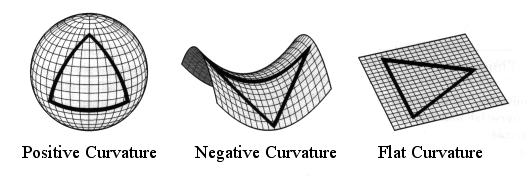All three geometries are classes of what is called Riemannian geometry, based on three possible states for parallel lines

• never meeting (flat or Euclidean)
• must cross (spherical)
• always divergent (hyperbolic)

or one can think of triangles where for a flat Universe the angles of a triangle sum to 180 degrees, in a closed Universe the sum must be greater than 180, in an open Universe the sum must be less than 180.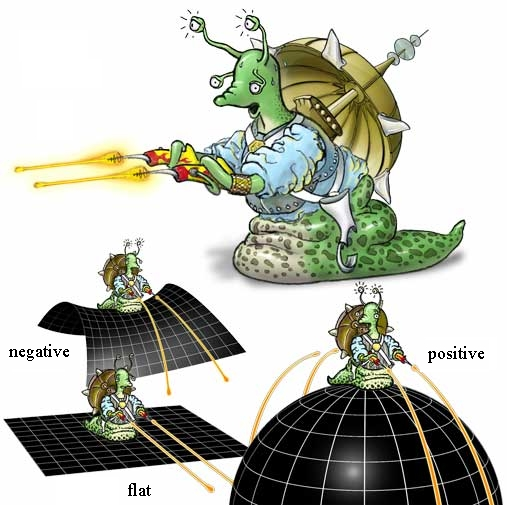Standard cosmological observations do not say anything about how those volumes fit together to give the universe its overall shape--its topology. The three plausible cosmic geometries are consistent with many different topologies. For example, relativity would describe both a torus (a doughnutlike shape) and a plane with the same equations, even though the torus is finite and the plane is infinite. Determining the topology requires some physical understanding beyond relativity.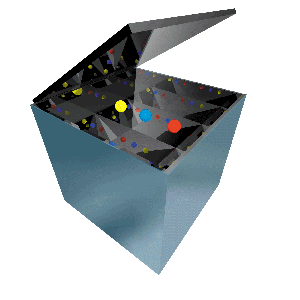Like a hall of mirrors, the apparently endless universe might be deluding us. The cosmos could, in fact, be finite. The illusion of infinity would come about as light wrapped all the way around space, perhaps more than once--creating multiple images of each galaxy. A mirror box evokes a finite cosmos that looks endless. The box contains only three balls, yet the mirrors that line its walls produce an infinite number of images. Of course, in the real universe there is no boundary from which light can reflect. Instead a multiplicity of images could arise as light rays wrap around the universe over and over again. From the pattern of repeated images, one could deduce the universe's true size and shape.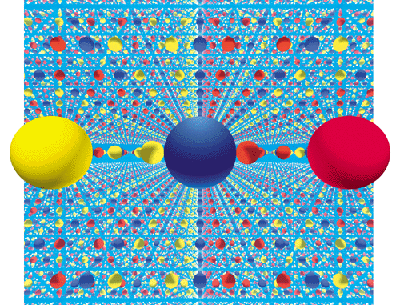Topology shows that a flat piece of spacetime can be folded into a torus when the edges touch. In a similar manner, a flat strip of paper can be twisted to form a Moebius Strip.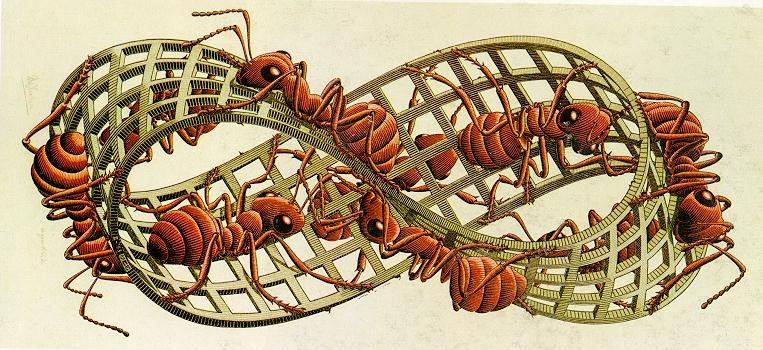The 3D version of a moebius strip is a Klein Bottle, where spacetime is distorted so there is no inside or outside, only one surface.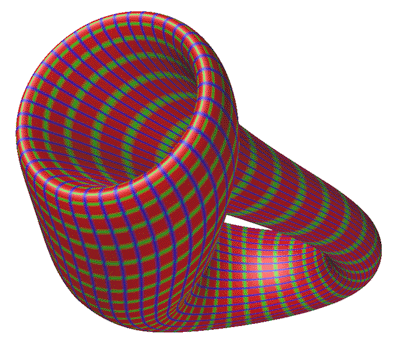The usual assumption is that the universe is, like a plane, "simply connected," which means there is only one direct path for light to travel from a source to an observer. A simply connected Euclidean or hyperbolic universe would indeed be infinite. But the universe might instead be "multiply connected," like a torus, in which case there are many different such paths. An observer would see multiple images of each galaxy and could easily misinterpret them as distinct galaxies in an endless space, much as a visitor to a mirrored room has the illusion of seeing a huge crowd.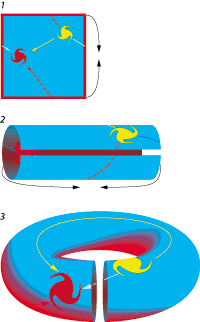One possible finite geometry is donutspace or more properly known as the Euclidean 2-torus, is a flat square whose opposite sides are connected. Anything crossing one edge reenters from the opposite edge (like a video game see 1 above). Although this surface cannot exist within our three-dimensional space, a distorted version can be built by taping together top and bottom (see 2 above) and scrunching the resulting cylinder into a ring (see 3 above). For observers in the pictured red galaxy, space seems infinite because their line of sight never ends (below). Light from the yellow galaxy can reach them along several different paths, so they see more than one image of it. A Euclidean 3-torus is built from a cube rather than a square.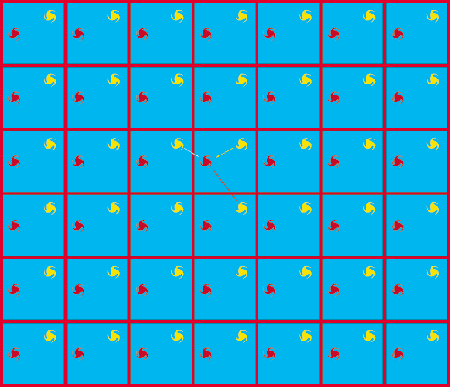A finite hyperbolic space is formed by an octagon whose opposite sides are connected, so that anything crossing one edge reenters from the opposite edge (top left). Topologically, the octagonal space is equivalent to a two-holed pretzel (top right). Observers who lived on the surface would see an infinite octagonal grid of galaxies. Such a grid can be drawn only on a hyperbolic manifold--a strange floppy surface where every point has the geometry of a saddle (bottom).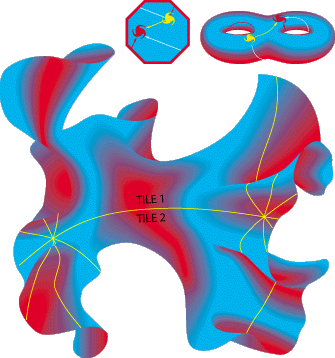Its important to remember that the above images are 2D shadows of 4D space, it is impossible to draw the geometry of the Universe on a piece of paper, it can only be described by mathematics. All possible Universes are finite since there is only a finite age and, therefore, a limiting horizon. The geometry may be flat or open, and therefore infinite in possible size (it continues to grow forever), but the amount of mass and time in our Universe is finite.

Measuring Curvature:

Measuring the curvature of the Universe is doable because of ability to see great distances with our new technology. On the Earth, it is difficult to see that we live on a sphere. One stands on a tall mountain, but the world still looks flat. One can see a ship come over the horizon, but that was thought to be atmospheric refraction for a long time.

Our current technology allows us to see over 80% of the size of the Universe, sufficient to measure curvature. Any method to measure distance and curvature requires a standard `yardstick', some physical characteristic that is identifiable at great distances and does not change with lookback time.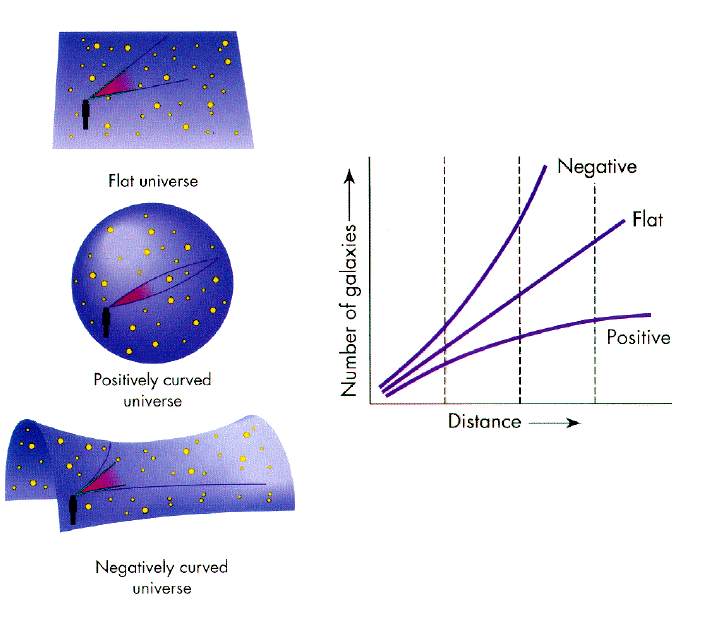The three primary methods to measure curvature are luminosity, scale length and number. Luminosity requires an observer to find some standard `candle', such as the brightest quasars, and follow them out to high redshifts. Scale length requires that some standard size be used, such as the size of the largest galaxies. Lastly, number counts are used where one counts the number of galaxies in a box as a function of distance.

To date all these methods have been inconclusive because the brightest, size and number of galaxies changes with time in a ways that we have not figured out. So far, the measurements are consistent with a flat Universe, which is popular for aesthetic reasons.